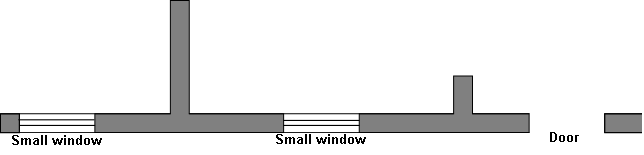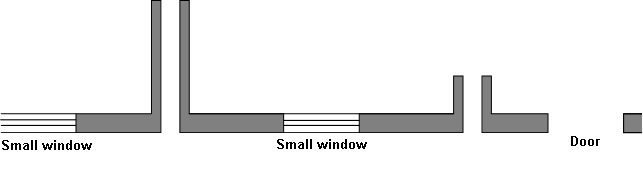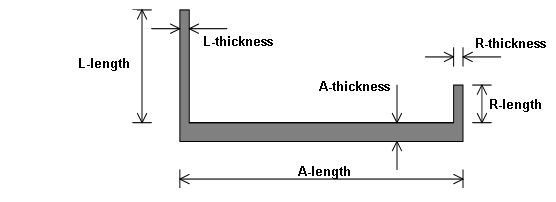#### Vertical loaded storey high walls of light weight concrete (EN 12602)

This module “Vertical loaded storey high walls of light weight concrete” is determining the load capacity for walls without openings. For a load bearing wall with large openings it will be necessary to determine the load capacity between openings individually. A load bearing wall with small openings can be considered as an integrated wall though. In both coincidences shall the total vertical load be distributed over the effective length of the wall (i.e. the sum of the wall sections between openings). No vertical load can be transferred through an opening (naturally).

Since the load is a combination of vertical load, horizontal load and moments of eccentricity, the load capacity is not a given value, but mn-diagrams. The given load-combination should be “inside” the mn-diagrams giving a sufficient load capacity.

A mn-diagram for a column gives the relation between a vertical load and a corresponding moment. For a column designed by a material without flexural strength there can’t exist a “moment” without a corresponding vertical load to stabilize the column.

The load-capacity is calculated in 2 states (the un-cracked and the cracked phase).

In the un-cracked phase the flexural strength is taking into consideration. The mn-diagram is developed from the extended Navier formula taking the column effect into consideration.

In the cracked phase the flexural strength is assumed to be zero. The mn-diagram is constructed from the Ritter formula. The load (actual value for m and n) should be inside only one of the curves (mn-diagram).

The actual combination of vertical load and moment is determined from:

• The vertical load on the top of the wall
• Eccentricity in top and bottom
• Out of plane deviation of the wall

The program determines combinations of vertical load and moment in different cross-sections and selects the cross-section with the largest moment as the design value. The combination of vertical load and moment is then compared with the mn-diagram’s.

#### Density

The value is transferred through the heading “LW Concrete (EN 12602)”. The value can be overwritten.

In several modules the dead load of the wall pr square meter are determined as the density multiplied the wall thickness. If any rendering should be added to the dead load the density should be corrected (e.g set 10 % higher to include the render). The thickness should be exact thus giving the “exact” load capacity.

#### Eccentricity e0

When determining the vertical load capacity, e0 is the eccentricity in top of wall (positive to the right). The users determine the total eccentricity in case the vertical loads consist of several contributions (e.g. slab to the left and right and a wall in top).

#### Characteristic modules of elasticity for the outer leaf E0k

The value is transferred through the heading “Masonry”. The value can be overwritten.

When calculating the ratio of slenderness consideration shall be taken to the total stiffness of the cavity wall. The stiffness of one leaf is proportional with t3 x E. Thus modules of elasticity and thickness shall be stated for both leafs

#### Out-of-plane eccentricity e5

When determining the vertical load capacity, e5 is the out-of-plane-eccentricity for the wall. The parameter assume a certain curvature of the wall (parabolic) and the value represent the maximum deflection in the middle. The parameter is not quite similar to einit in EN 1996 - 1 - 1, where the deflection einit is in the full height.

#### Module of elasticity for the bearing wall (Inner leaf) Eck

The value is transferred through the heading “LW Concrete (EN 12602)”. The value can be overwritten.

The module of elasticity is used, when determining the column-factor ks

#### Eccentric reaction at the footing

The support condition at the footing can be regarded as simple (no moment can be transferred = equal to nil eccentricity) or with an eccentric reaction giving a moment in favour for the construction. The eccentricity is t/6. The actual value is determined through an elastic calculation.

#### Declared compressive strength fck

The value is transferred through the heading “LW Concrete (EN 12602)”. The value can be overwritten.

#### Declared flexural strength fcflk

The value is transferred through the heading “LW Concrete (EN 12602)”. The value can be overwritten.

#### Height

If the wall is placed on a slab or a foundation, the height is measured from the top of the slab or foundation.

If the wall at the top is horizontal supported by a floor component, resting on the upper side of the wall, the design value of the height is measured to the lower side of the floor component.

The assumptions for the calculation in this module are a wall supported at the top and bottom and without openings. The vertical load at the top of the wall shall be corrected for openings if any. This shall be done geometrically. E.g for a 6,0 m long wall with 2 openings each with a width of 1,0 m the vertical load shall be increased a factor: 6/(6-4) = 1,5. This correction gives the correct load in the middle height where the failure will occur. The vertical load can normally be extracted from the module “calculation of loads”

The dead load of the wall shall not be implemented in the value. The dead load is calculated via the density and thickness

A vertical point load can be distributed in 1:2. The height of the wall is e.g 2,8 m. A point load at the top on the central part of the wall (central part = minimum 2,8 m / 4 = 0,7 m horizontal from a vertical edge) can be regarded as having a horizontal extension for the wall of: 2 x 1,4 m/2 = 1,4 m.

Is the concentrated load direct on the vertical edge the horizontal extension for the wall can be estimated to: 1 x 1,4 m/2 = 0,7 m.

In both situations vertical split-forces shall be thoroughly examinated

This load is practically always wind load, either directly acting on the exposed wall or through the evenly distributed wall-ties. The value is always positive (going to the left). The eccentricity for the vertical load can be positive or negative and thus acting in the same or opposite direction of the wind load.

If the capacity of the outer leaf is used for stiffening the load bearing inner wall the total windload shall be applied on the inner wall

#### Thickness. Outer leaf

When calculating the ratio of slenderness consideration shall be taken to the total stiffness of the combined wall. The stiffness of one leaf is proportional with t3 x E. Thus modules of elasticity and thickness shall be stated for both leafs

The thickness should be reduced in case joints are recessed.

Rules in the N.A. for minimum thickness of walls shall be applied

#### Type of wall

According to EN 12602 shall the ratio of slenderness (leff/ic ) shall be smaller or equal to 120. For rectangular cross sections this is equivalent to leff/teff < 34,6

For single-leaf wall teff is the effective thickness (deducted recessed joints if any)

For cavity wall teff is calculated according to the cubic – formula taking into consideration the stiffness of the outer leaf through its thickness and modules of elasticity

#### Horizontal dimensions

The effective height is reduced by the program when the wall has one or two vertical supports in the ends.

These supporting walls consist typically of walls perpendicular to the wall regarded and they can support more than the actual wall, typically a continuation of the actual wall. These supporting walls can be split arbitrarily between the walls they support. See sketches below.Figure 2. ConstructionFigure 3. Split of vertical supporting wallsFigure 4. Designed wallFigure 5. Designations

Here:

• L is the supporting wall to the left
• R is the supporting wall to the right
• A is the actual wall to be supported